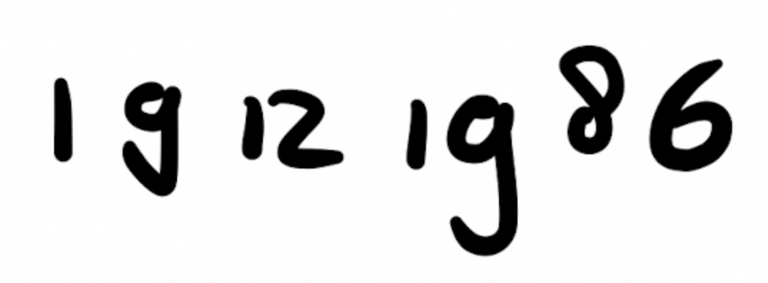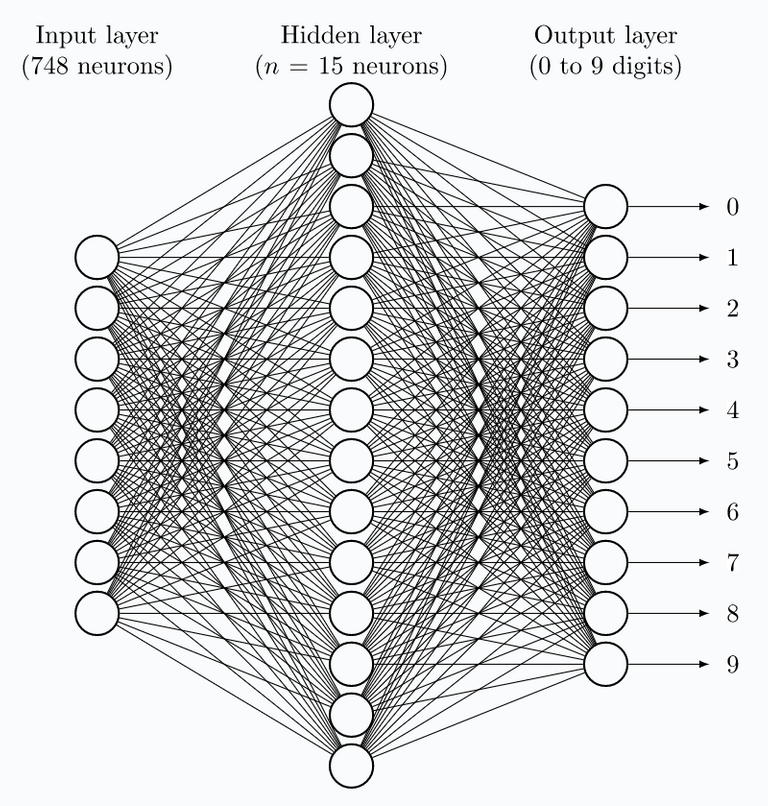Handwritten Digit RecognitionThis post will focus on how a neural network can be used to identify handwritten digits. This is a very popular introductory problem to neural networks. You could call it the "Hello World!" problem for the field of neural networks.

This is the third post in a series on neural networks and deep learning. This series is my attempt to get more familiar with the topic and is heavily based on the book by Michael Nielsen.

This network to classify handwritten digits will take digital scans of single digit numbers. The grayscale, 28 by 28 pixel images of scanned handwritten digits are used as input to a neural network of which the output is a number between 0 and 9.The greyscale value between 0.0 and 1.0 of each pixel is used as an input to the input layer of neurons. Hence, the input layer consists of 28×28=784 neurons. In this problem, a white pixel corresponds the a 0.0 input value and black to an input value of 1.0.

A simplified sketch of this network is shown below. For simplicity the input layer is showing only 8 input neurons. The number of neurons in the hidden layer shown in the figure is set to 15. The number of neurons in the hidden layer can be altered to give different results. In a later post discussing the implemented network, will show different number of hidden layer nodes.The neuron in the output layer with the highest activation value represents the digit in the given image. E.g., when the 5th neuron of the output layer is firing, the given input image is likely showing the number 4.

In order to make a network do what we want, which in our case means classifying images of digits, we need to train the network in order for it to do a good job. In the next post we will be looking at how this can be done.